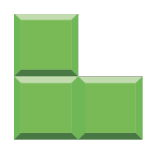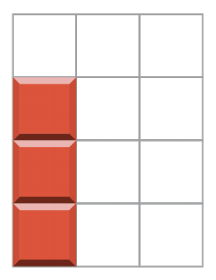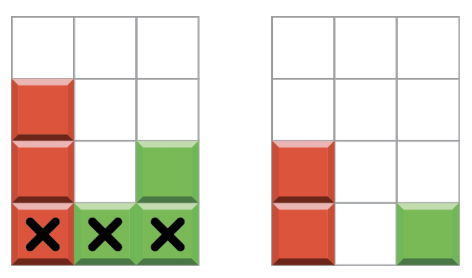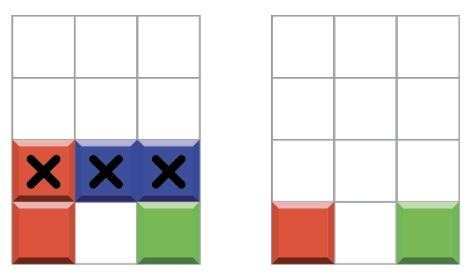시간 제한메모리 제한제출정답맞힌 사람정답 비율
2 초 512 MB97777.778%

## 문제

As you may know, the popular computer game “Tetris” was invented by Russian programmer Alexey Pajitnov. In this problem you need to write program which plays a simplified version of this game.

The playing field is a rectangular vertical shaft, called the “well”. Random figures of unit square blocks appear on top of the wall, the player chooses the horizontal position and rotation of the figure, after that the figure falls down in the well. The objective of the game is to create horizontal lines filled without gaps. When such a line is created, it disappears, and any blocks above the deleted line fall.

In this version of the game, the well size units, and there are only three types of figures:

Type Figure
123You lose if at some point there are five non-empty lines of the well. You win if you hadn't lost after n figures have fallen.

You need to write program which plays the game described above and wins regardless of which figures will appear.

## 구현

You should implement four functions (methods):

• void init(int n). This function is called before any other function.
• void new_figure(int figure_type). This function is called when a new figure appears. figure_type is a number from 1 to 3, indicating the type of the figure according to the table above.
• int get_position(). This function should return a number from 0 to 2, the position of the leftmost block of the last figure.
• int get_rotation(). This function should return a number from 0 to 3, the number of time the figure was rotated couter-clockwise.

Functions get_position and get_rotation will be called only after new_figure.

## 예제

The grader makes the following function calls:

• init(3). There will be three figures.
• new_figure(1). A figure of type 1 falls from the top of the well.
• get_position() returns 0. This means that player wants to put the figure in the leftmost column.
• get_rotation() returns 1 (or 3). This means that player wants to rotate the figure vertically.
• After the figure has fallen, the well looks like this:• new_figure(3).
• get_position() returns 1.
• get_rotation() returns 1.
• After the figure has fallen, the first line is full, so it disappears and the well looks like this:• new_figure(2).
• get_position() returns 1.
• get_rotation() returns 0 (or 2).
• After the figure has fallen, the second line is full, so it disappears and the well looks like this:## 제한

In all subtasks n ≤ 1000.

## 서브태스크

번호배점제한
17

All figures have type 1.

28

All figures have type 2.

310

All figures have type 1 or 2.

421

All figures have type 3.

554

Figures can have different types.

## 샘플 그레이더

The sample grader reads the input in the following format:

• Line 1: One integer n.
• Line 2: n integers: types of figures.

## 제출할 수 있는 언어

C++17, C++14, C++20, C++14 (Clang), C++17 (Clang), C++20 (Clang)

## 채점 및 기타 정보

• 예제는 채점하지 않는다.